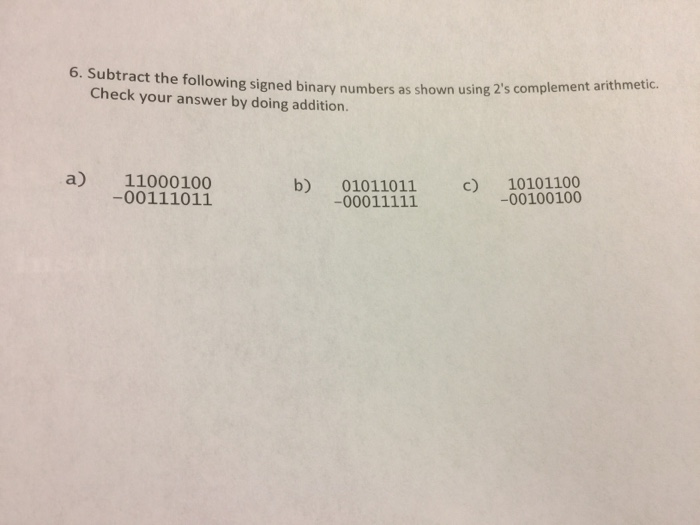# Question & Answer: Subtract the following un-signed binary numbers as shown using 2's complement arithmetic. Check your answer by…..Subtract the following un-signed binary numbers as shown using 2’s complement arithmetic. Check your answer by doing addition. a) 11000100 -00111011 b) 01011011 -00011111 c)10101100 -00100100

Don't use plagiarized sources. Get Your Custom Essay on
Question & Answer: Subtract the following un-signed binary numbers as shown using 2's complement arithmetic. Check your answer by…..
GET AN ESSAY WRITTEN FOR YOU FROM AS LOW AS \$13/PAGE

a)11000100 – 00111011=10001001

in decimal it is 137.

b)01011011 – 00011111 =111100 (or)00111100

in decimal it is 60.

c)10101100 – 00100100 =10001000

in decimal 136